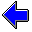Java Security Settings:
This web page employs Java, which requires specific security settings for correct operation.
If the applets on this page do not run correctly, consult the Virtual Chemistry Experiments FAQ
or the Physlet Physics web site for establishing the correct security settings.

# Virtual Chemistry Experiments

## Atomic Orbitals

Topic Concepts Experiment
Atomic Orbitals
Atomic Orbitals (single window)
Isosurfaces of various atomic orbitals and some hybrid orbitals are displayed.
Orbitals Isosurfaces, radial distribution plots, and electron density plots of various atomic orbitals are displayed.
Graphical Representations of Orbitals The quantum numbers for hydrogen orbitals are explained. Three ways of representing orbitals (radial distribution, electron density, and isosurface plots) are described. A radial distribution plot, an electron density plot, and a virtual reality isosurface plot for the hydrogen 1s orbital are displayed to illustrate the significance and use of each type of orbital representation.
s Orbitals The quantum numbers for s orbitals are identified. The shape of s orbitals is explored. For s orbitals with various principal quantum numbers, radial distribution, electron density, and isosurface plots are examined to determine the number and shapes of nodal surfaces and the region where the electron is most likely to be found.
p Orbitals The quantum numbers for p orbitals are identified. The shape and orientations of p orbitals are explored. For p orbitals with various principal quantum numbers, radial distribution, electron density, and isosurface plots are examined to determine the number and shapes of nodal surfaces.
d Orbitals The quantum numbers for d orbitals are identified. The shape and orientations of d orbitals are explored. For d orbitals with various principal quantum numbers, radial distribution, electron density, and isosurface plots are examined to determine the number and shapes of nodal surfaces.
Sizes of Atomic Orbitals The shell designations for atomic orbitals are presented. A virtual reality isosurface display is used to compare sets of atomic orbitals with various quantum numbers. This display allows direct comparison of the sizes of the orbitals.
Effective Nuclear Charge The effect of shielding and the concept of an effective nuclear charge are explained. A virtual reality isosurface display is used to compare the sizes of hydrogen 1s and 2s orbitals with various effective nuclear charges with the sizes of fluorine 1s and 2s orbitals.
Effective Nuclear Charge and Orbital Size Slater rules for predicting shielding factors and Zeff are introduced. A virtual reality isosurface display is used to compare the sizes of a hydrogen s orbital with a user-defined Zeff and an s orbital of a multi-electron atom. The best Zeff for the s orbital of the multi-electron atom is determined by matching the sizes of the orbitals and this value is compared with that predicted by the Slater rules.
Hybrid Orbitals The origin and significance of hybrid orbitals is explained and illustrated with electron density plots and energy diagrams.
Geometry of Hybrid Orbitals The purpose of hybrid orbitals is explained. Small balls are arranged in a linear, trigonal planar, or tetrahedral geometry. Various hybrid orbitals may be displayed and oriented towards the balls. Only the proper hybridization scheme will provide a set of orbitals that can simultaneously be directed at all of the balls.

###Virtual Chemistry Experiments Home Page

© Copyright 2001, 2008, David N. Blauch
Last updated Tuesday April 22 2014